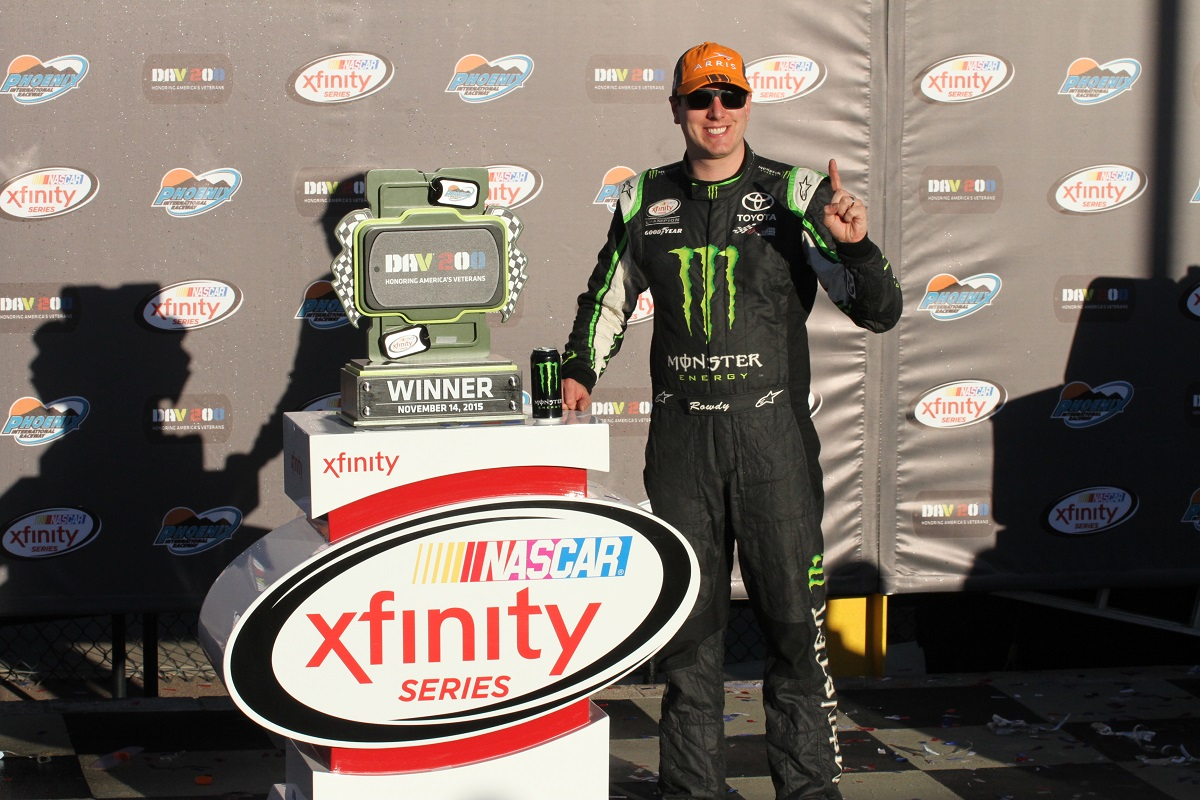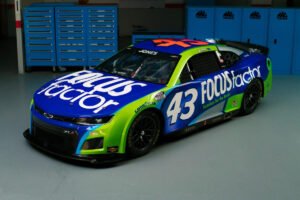December 1, 2021

# OnPitRoad.com Pick ‘Em Fantasy Pick Results for Dav 200 Honoring America’s VeteransThe Picks

Ronald Costigan picked Chase Elliott, Kyle Busch and Kasey Kahne. Elliott finished seventh with Busch winning and Kahne 10th. Points: 37+48+34=119

Ashley McCubbin picked Chase Elliott, Erik Jones and Kyle Busch. Jones finished third. Points: 37+48+41=126

Justin Tucker picked Erik Jones, Brad Keselowski and Kyle Busch. Keselowski finished 2nd. Points: 41+43+48=132

Robert Hill picked Darrell Wallace Jr., Erik Jones and Ryan Sieg. Wallace finished eighth with Sieg 16th. Points: 36+48+28=112

Tim Johnston, Marshal Gabell and Brooke Clarke picked Chase Elliott, Brad Keselowski and Kasey Kahne. Points: 37+43+34=114

Kreistina Blinova picked Ty Dillon, Austin Dillon and Kasey Kahne. Ty finished fifth and Austin 14th. Points: 39+30+34=103

Kyle Magda picked Ruben Pardo, Brad Keselowski and Daniel Suarez. Pardon finished 38th with Suarez fourth. Points: 6+43+40=89

Steve Love picked Regan Smith, Brad Keselowski and Kyle Larson. Smith finished sixth with Larson 15th. Points: 38+43+29=110

Jeni Harris picked Chase Elliott, Erik Jones and Ross Kenseth. Kenseth finished 30th. Points: 37+48+11=96

Doug Townsend picked Brad Keselowski, Kyle Busch and Chris Buescher. Buescher finished 13th. Points: 43+48+31=122

Brandon Feeney picked Ty Dillon, Kyle Busch and Kasey Kahne. Points: 39+48+34=121

Parker Ledger picked Regan Smith, Chase Elliott and Kasey Kahne. Points: 38+37+34=109

Rory Townsend picked Chase Elliott, TJ Bell and Kasey Kahne. Bell finished 36th. Points: 37+8+34=79

Alex George picked Chase Elliott, Kyle Larson and Kasey Kahne. Points: 37+29+34=100

Mike Cousins picked Ty Dillon, Chris Buescher and Kasey Kahne. Points: 39+31+34=104

Jeffrey Breazeale picked Regan Smith, Brad Keselowski and Kyle Busch. Points: 38+43+48=129

Stephen Placido picked Darrell Wallace Jr., Brad Keselowski and Kasey Kahne. Points: 36+43+34=113

The Results

1. Justin Tucker – 132
2. Jeffrey Breazeale – 129
3. Ashley McCubbin – 126
4. Doug Townsend – 122
5. Brandon Feeney – 121
6. Ronald Costigan – 119
7. Tim Johnston, Marshall Gabell and Brooke Clarke – 114
8. Stephen Placido – 113
9. Robert Hill – 112
10. Steve Love – 110
11. Parker Ledger – 109
12. Mike Cousins – 104
13. Kreistina Blinova – 103
14. Alex George – 100
15. Jeni Harris – 96
16. Kyle Magda – 89
17. Rory Townsend – 79

Current Fall XFINITY Series Standings

1. Justin Tucker – 690+132=822
2. (Points Leader Post Chicago) Steve Love – 704+110=814 (-8)
3. Jeffrey Breazeale – 684+129=813 (-9)
4. Brandon Feeney – 676+121=797 (-25)
5. Ronald Costigan – 673+119=792 (-30)
6. Doug Townsend – 667+122=789 (-33)
7. Ashley McCubbin – 653+126=779 (-43)
8. Jeni Harris – 656+96=752 (-70)
9. Parker Ledger – 633+109=742 (-80)
10. Robert Hill – 626+112=738 (-84)
11. Tim Johnston, Marshall Gabell and Brooke Clarke – 624+114=738 (-84)
12. Kreistina Blinova – 632+103=735 (-87)
13. Mike Cousins – 615+104=719
14. Stephen Placido – 604+113=717
15. Alex George – 556+100=656
16. Kyle Magda – 517+89=606
17. Rory Townsend – 464+79=543

Full Season XFINITY Series Standings

2. Ronald Costigan – 3529+119=3648 (-70)
3. Jeffrey Breazeale – 3491+129=3620 (-88)
4. Ashley McCubbin – 3470+126=3596
5. Brandon Feeney – 3438+121=3559
6. Doug Townsend – 3411+122=3533
7. Steve Love – 3403+110=3513
8. Brooke Clarke – 3296+114=3410
9. Tim Johnston – 3288+114=3402
10. Stephen Placido – 3278+113=3391
11. Kyle Magda – 3224+89=3313
12. Mike Cousins – 3191+104=3295
13. Parker Ledger – 3177+109=3286
14. Marshall Gabell – 3166+114=3280
15. Kreistina Blinova – 3165+103=3268
16. Jeni Harris – 3123+96=3219
17. Robert Hill – 3106+112=3218
18. Alex George – 2960+100=3060
19. Rory Townsend – 2787+79=2866

Fall Overall Standings

1. (Points Leader Post Cup Martinsville) Steve Love – 1973+70+110=2153
2. Doug Townsend – 1952+69+122=2143 (-10)
3. Brandon Feeney – 1876+84+121=2081 (-72)
4. Justin Tucker – 1865+59+132=2056 (-97)
5. Parker Ledger – 1862+77+109=2048
6. Jeni Harris – 1868+77+96=2041
7. Ashley McCubbin – 1836+55+126=2017
8. Ronald Costigan – 1823+55+119=1997
9. Brooke Clarke – 1828+52+114=1994
10. Mike Cousins – 1789+69+104=1962
11. Kreistina Blinova – 1801+52+103=1956
12. Tim Johnston – 1768+65+114=1947
13. Stephen Placido – 1745+52+113=1910
14. Marshall Gabell – 1707+65+114=1886
15. Jeffrey Breazeale – 1679+57+129=1865
16. Alex George – 1692+42+100=1834
17. Rory Townsend – 1650+66+79=1795
18. Robert Hill – 1431+78+112=1621
19. Kyle Magda – 1523+67+89=1379

Full Season Overall Points Standings

1. (Points Leader Post Cup Kansas) Justin Tucker – 8631+59+132=8822
2. Ronald Costigan – 8454+55+119=8628 (-194)
3. Ashley McCubbin – 8400+55+126=8581
4. Brandon Feeney – 8359+84+121=8564
5. Doug Townsend – 8362+69+122=8553
6. Steve Love – 8309+70+110=8489
7. Stephen Placido – 8178+52+113=8343
8. Parker Ledger – 8149+77+109=8335
9. Brooke Clarke – 8080+52+114=8246
10. Tim Johnston – 8041+65+114=8220
11. Mike Cousins – 8014+69+104=8187
12. Jeffrey Breazeale – 7931+57+129=8118
13. Kreistina Blinova – 7959+52+103=8107
14. Marshall Gabell – 7842+65+114=8021
15. Kyle Magda – 7847+67+89=8003
16. Jeni Harris – 7823+77+96=7996
17. Alex George – 7792+42+100=7934
18. Rory Townsend – 7497+66+79=7642
19. Robert Hill – 6953+78+112=7143

#### You may have missed#### Why Progressive Insurance is the right choice#### FOCUSFACTOR® TO PARTNER WITH RICHARD PETTY MOTORSPORTS#### Spire Motorsports to Field NASCAR Camping World Truck Series Team in 2022